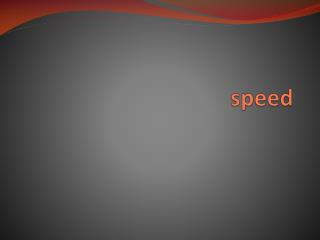DownloadDownload Presentationspeed

# speed

Download Presentation## speed

- - - - - - - - - - - - - - - - - - - - - - - - - - - E N D - - - - - - - - - - - - - - - - - - - - - - - - - - -
##### Presentation Transcript

1. speed

2. Speed: • The speed of something is how fast it is moving. • Speed is measured in: • Metres per second: ms-1 • Kilometres per hour: km/h or kmh-1 • The formula for speed is: v=d/t • v: means speed, the other name for speed is velocity • d: is distance in metres • t: is time in seconds

3. E.g. Maureen and Megan studied whether cars passing their school were breaking the speed limit. • The speed limit outside the school was 50 km/h (14 ms-1 ). They measured 70m. Maureen waved as cars crossed a starting line on the road and Megan used a stopwatch to time how long it took them to cross the line in front of her. Any car that travelled the 70 m in less than 5 s was speeding. • v=d/t using this formula, complete the table on the next screen

4. Acceleration and deceleration

5. acceleration • Unbalanced forces produce a changing motion. If it speeds up the object, it is accelerating. • Acceleration is measured in metres per second per second: ms-2 . In other words, how much faster it goes in metres per second every second. • a=v/t. F=ma. • There are 2 formulas that have acceleration in them. • Acceleration = speed/time • Force = mass X acceleration

6. Deceleration: • Deceleration is the slowing down of an object. We show deceleration by putting a negative sign in front of the number: • E.g. a car decelerates at -10 ms-2 .

7. Changing equations:

8. Changing equations • If we put the equation into a triangle, we can easily work out the other equations. • We put our finger over the thing we are trying to work out and then write down the equation. • v=d/t; • d=vt; • t=d/v d v t